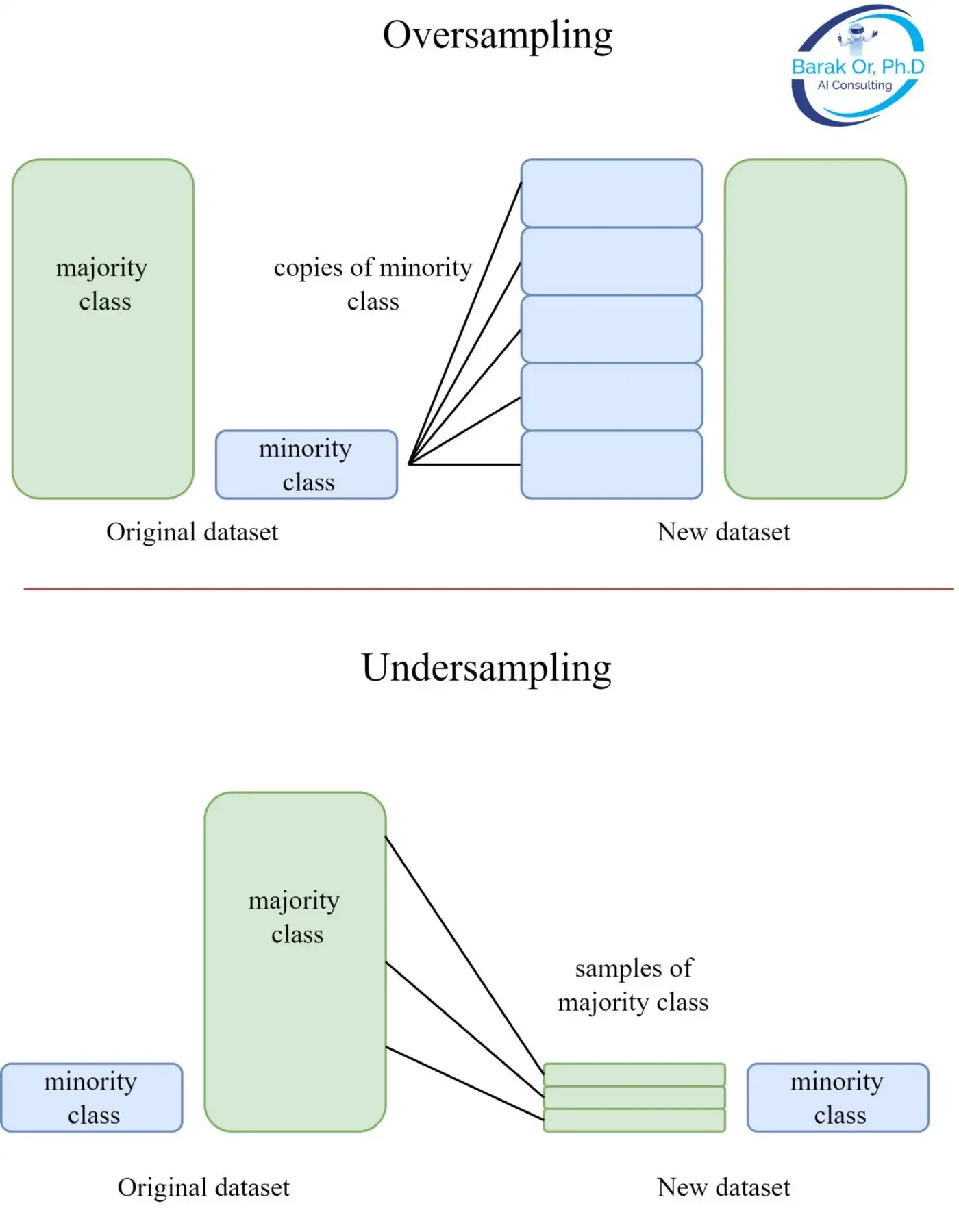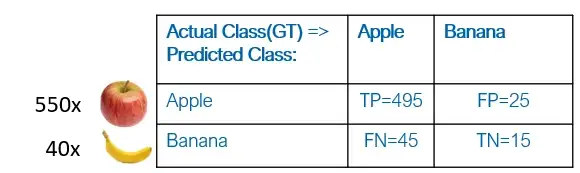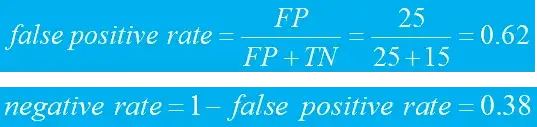top of page
•Barak Or

# Solving The Class Imbalance Problem

Class imbalance is a common issue where the distribution of examples within a dataset is skewed or biased.

Introduction

Imbalanced classification is a common problem in machine learning, particularly in the realm of binary classification. This occurs when the training dataset has an unequal distribution of classes, leading to a potential bias in the trained model. Examples of imbalanced classification problems include fraud detection, claim prediction, default prediction, churn prediction, spam detection, anomaly detection, and outlier detection. It is important to address the class imbalance in order to improve the performance of our model and ensure its accuracy.

Notice that most, if not all, of the examples, are likely binary classification problems. So, Imbalance is Common!

In this post, we will examine three methods for addressing this problem in order to improve the performance and accuracy of our models. We will also discuss the importance of choosing the right metric for these types of tasks.From multi-class to bi-class

We will cover the concept of binary classification and how it can be utilized to address the challenges of class imbalance. Binary classification involves dividing a dataset into two groups: a positive group and a negative group. These principles can also be extended to multi-class problems by decomposing the problem into multiple two-class problems. This technique allows us to address class imbalance and utilize a range of methods to enhance the performance of our model.

Common practice

There are several methods that can be used to address class imbalance in machine learning. One approach is undersampling or oversampling, also known as “class augmentation,” which involves adjusting the number of samples in the minority or majority class to improve the balance of the dataset. Another option is to change the weights on the loss function, which can help the model focus more on the minority class during training. Finally, it is possible to initialize the bias of the final layer to predict unequal probabilities, allowing the model to better predict the minority class. These approaches can be used individually or in combination, depending on the needs of the specific problem.

Under/Over Resampling

Resampling is a common technique used to address class imbalance in machine learning. It involves creating a new version of the training dataset with a different class distribution by selecting examples from the original dataset. One popular method of resampling is random resampling, where examples are chosen randomly for the transformed dataset. Resampling is often considered a simple and effective strategy for imbalanced classification problems because it allows the model to more evenly consider examples from different classes during training. However, it is important to carefully consider the trade-offs and limitations of resampling, as it can also introduce additional noise and bias into the dataset. The picture below provides illustrations for oversampling (upper) and undersampling (lower).Weights modification on a loss function

The second method for addressing class imbalance is to modify the weights on the loss function. In a balanced dataset, the gradient of the loss function (i.e., the direction towards the local minimum) is calculated as the average gradient for all samples.However, in an imbalanced dataset, this gradient may not accurately reflect the optimal direction for the minority class. To address this issue, we can decompose the gradient by either oversampling as a part of the optimization process or by using a weighted loss.Oversampling involves artificially increasing the number of minority class examples in the dataset, which can help the model more accurately consider those examples during training.Alternatively, using a weighted loss involves assigning higher weights to the minority class examples, so that the model places more emphasis on correctly classifying those examples.Both of these methods can help improve the performance of the model on imbalanced datasets.

Bias Initialization

The last technique we introduce in this post for addressing class imbalance in machine learning is bias initialization, which involves adjusting the initial values of the model’s parameters to better reflect the distribution of the training data. More specifically, we will set the final layer bias. For example, in an imbalanced binary classification problem with a softmax activation function, we can set the initial bias of the final layer to be b=log(P/N), where P is the number of positive examples and N is the number of negative examples. This can help the model more accurately measure the probability of the positive and negative classes at the initialization of the training process, improving its performance on imbalanced datasets.

It is important to carefully consider the trade-offs and limitations of bias initialization, as it can potentially introduce additional bias into the model if you initialize it wrong. However, when used properly, this technique can be an effective and efficient way to address class imbalance and improve the performance of the model.

Classification metrics

When working with imbalanced datasets in machine learning, it is crucial to choose the right evaluation metrics in order to accurately assess the performance of the model. For example, in a dataset with 99,000 images of cats and only 1,000 images of dogs, the initial accuracy of the model might be 99%. However, this metric may not provide a true representation of the model’s ability to accurately classify the minority class (dogs).

One useful tool for evaluating the performance of a classifier on imbalanced datasets is the confusion matrix-based metrics. This matrix provides a breakdown of the true positive, true negative, false positive, and false negative predictions made by the model, allowing for a more nuanced understanding of its performance. It is important to consider a variety of metrics when evaluating a model on imbalanced datasets in order to get a comprehensive understanding of its capabilities.

A quick review of the confusion matrix: In evaluating the performance of a classifier, it is helpful to consider a variety of metrics. A confusion matrix is a useful tool for understanding the true positive (TP) predictions, where the model correctly identified the positive class, as well as the false negative (FN) predictions, where the model incorrectly classified a sample as the negative class that was actually positive. The confusion matrix also provides information on false positive (FP) predictions, where the model incorrectly identified a sample as the positive class that was actually negative, and true negative (TN) predictions, where the model correctly identified the negative class. By considering these different types of predictions, we can gain a more comprehensive understanding of the model’s performance.In order to understand the performance of a classifier, it is important to consider a range of evaluation metrics. Accuracy, precision, and recall are three commonly used metrics that can be calculated from the confusion matrix.

Accuracy reflects the over all accuracy of the model’s predictions, calculated as the number of correct predictions divided by the total number of predictions. Precision measures the proportion of positive predictions that were actually correct, calculated as the number of true positive predictions divided by the total number of positive predictions made by the model. And recall, also known as sensitivity or true positive rate, captures the proportion of actual positive samples that were correctly predicted by the model, calculated as the number of true positive predictions divided by the total number of actual positive samples.An example of classifying apples and bananas (90:10):In this example, the metrics may indicate strong performance for the apple class. However, it is important to also consider the performance of the banana class, as the model’s overall performance may not be uniformly strong. It is still necessary to assess the model’s performance on the banana class in order to fully understand its capabilities. By considering the performance of both classes, we can identify any potential imbalances for improvement in the model. We will use two additional metrics, the false positive rate, and the negative rate. The false positive rate represents the proportion of actual negative samples that were incorrectly predicted as positive by the model, calculated as the number of false positive predictions divided by the total number of actual negative samples. The false negative rate reflects the proportion of actual positive samples that were incorrectly predicted as negative by the model, calculated as the number of false negative predictions divided by the total number of actual positive samples.In this case, it is clear that there is an imbalanced class problem. Detecting and diagnosing class imbalance can be challenging, and it is important to use the appropriate metrics in order to identify it.

Summary

Class imbalance is a common problem in machine learning that occurs when the distribution of examples within a dataset is skewed or biased. This can lead to a bias in the trained model, which can negatively impact its performance. In this post, we explored various methods for addressing class imbalance, including resampling, modifying the weights on the loss function, and initializing the bias of the final layer. These techniques can be utilized individually or in combination. We also emphasized the importance of selecting the right evaluation metric, such as accuracy, precision, and recall, to accurately assess the performance of these models. By understanding and addressing class imbalance, we can greatly improve the reliability and effectiveness of our models.

More resources:

 A Gentle Introduction to Imbalanced Classification by Jason Brownlee

 Guide to Classification on Imbalanced Datasets by Matthew Stewar

 Imbalanced Data: an extensive guide on how to deal with imbalanced classification problems by Lavina Guadagnolo

 Understanding AUC — ROC and Precision-Recall Curves by Maria Gusarova

 Dealing with Imbalanced Data by Tara Boyle

 Is F1 the appropriate criterion to use? What about F2, F3,…, F beta? By Barak Or

צפייה 10 תגובות
bottom of page